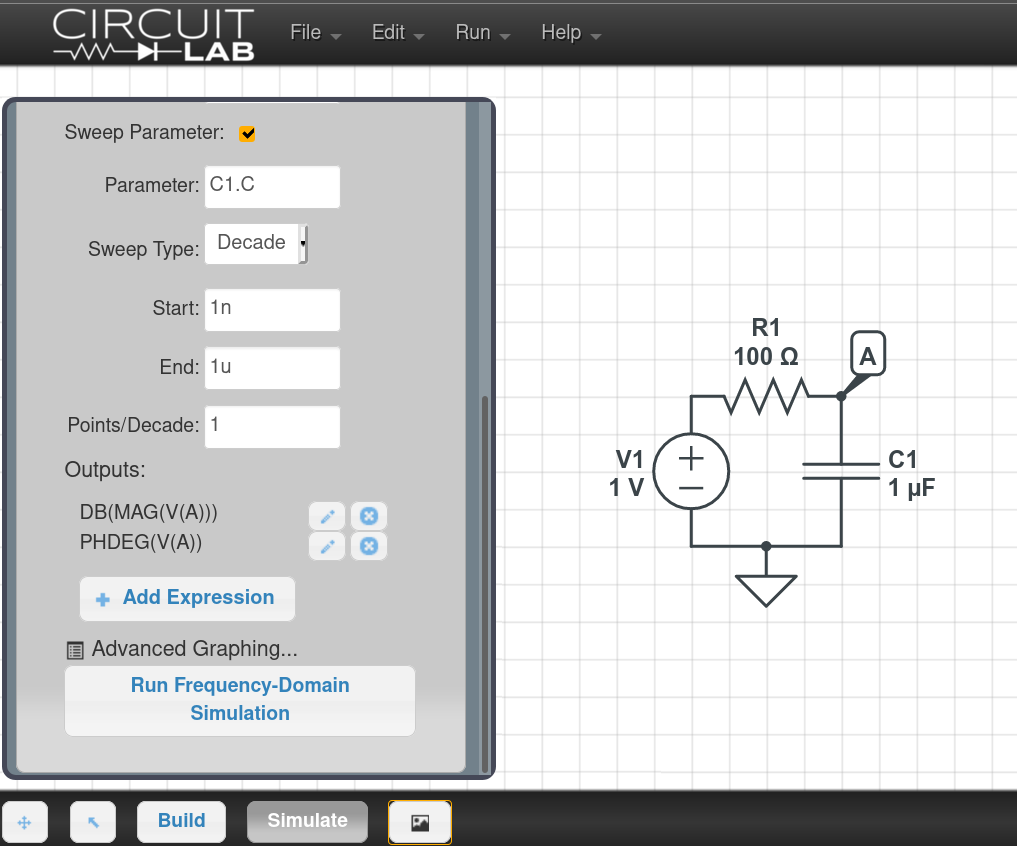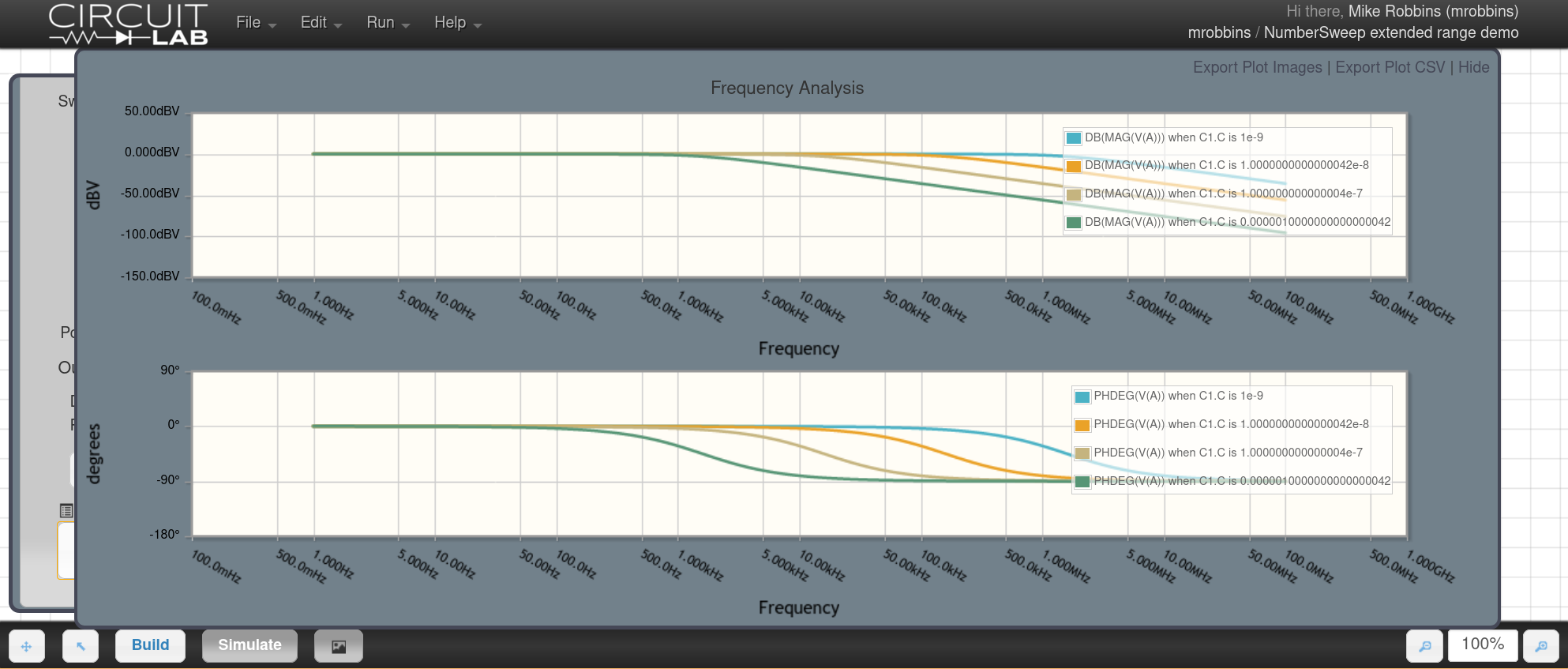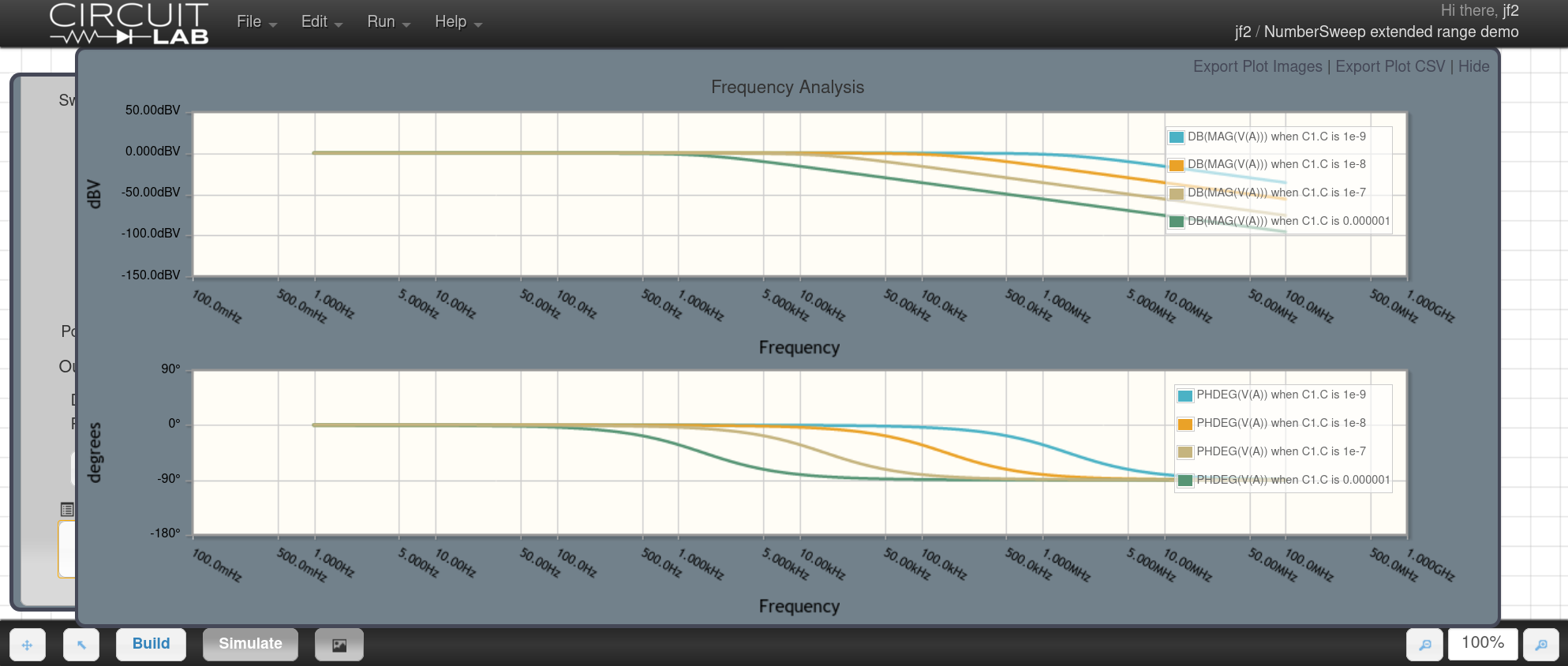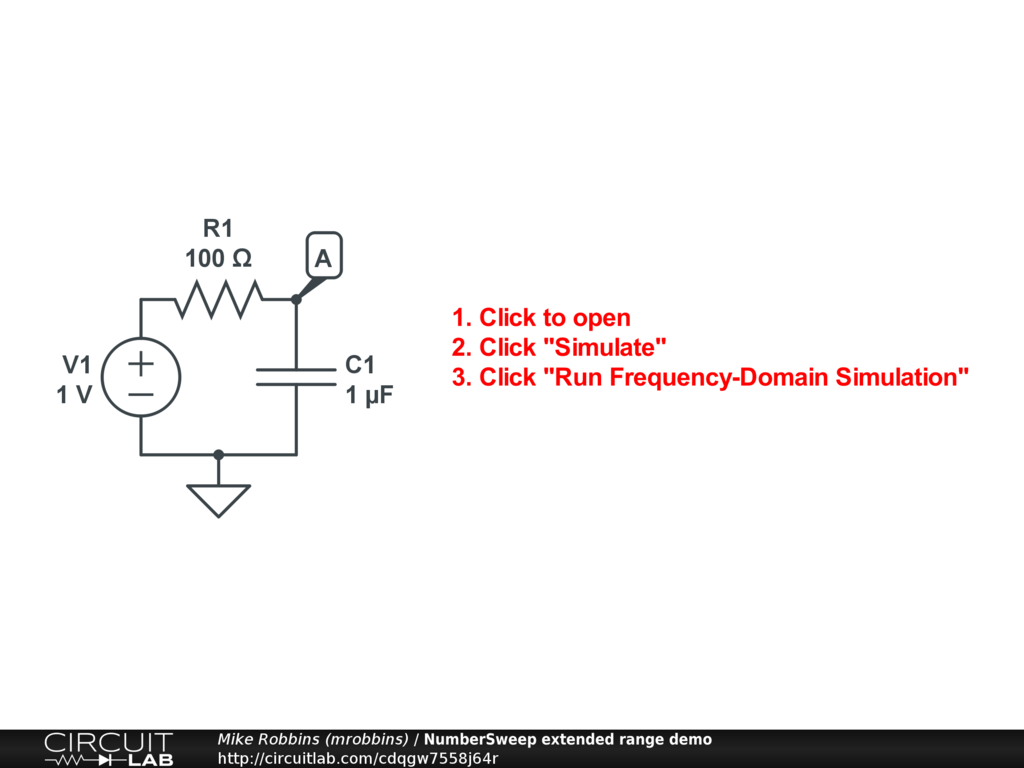## Extended Numerical Precision for Parameter Sweeps

#### Jan 04 2021, 1:00 PM PST · 0 comments »

CircuitLab has always made it easy to simulate the same circuit with variations of one or more parameters. For example, we can quickly compare the frequency response (Bode plot) of a RC low-pass filter with different capacitors by setting up the simulation with a Decade sweep over parameter C1.C from 1n to 1u:With 1 point per decade, this instructs the circuit simulator to build the circuit with four values for capacitor $C_1$, specifically $1 \ \text{nF}, 10 \ \text{nF}, 100 \ \text{nF}, 1 \ \mu\text{F}$. The four responses are compared by plotting them in different colors on a single Bode plot. With old CircuitLab software prior to today, the result looked like this:While it works, you’ll notice in the plot legend that there are rounding errors in the values, such as when C1.C is 1.0000000000000042e-8, when it should be precisely 1e-8. That happens because the computer represents values as 64-bit floating point, leading to roughly 16 decimal digits of precision.

Instead of simply rounding or truncating the display, we’ve fixed this by applying our extended numerical precision to parameter sweeps as well. See our earlier article Double-Double, Please! When 64-Bit Floating Point Isn’t Enough for more details about extended precision numerical routines.

As of today, this issue has been fixed, and the capacitor values are calculated precisely:We hope this makes CircuitLab a more pleasant tool to use for investigating circuit behavior.

Try the example simulation for yourself: